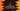# C program to find the sum of 'n' numbers using dynamic memory allocation### Write a C program to find the sum of ‘n’ numbers using dynamically allocating memory :

In this tutorial, we will learn how to find the sum of ‘n’ numbers by allocating memory dynamically. Allocating memory dynamically means we will use only the amount of memory that is required. If user will enter 10 numbers, we will allocate for 10 numbers. Similarly, for 100 numbers, we will allocate for 100 numbers. To allocate the memory, we will use malloc method. Let’s take a look into the program first :

### C program to allocate memory dynamically and find the sum of ‘n’ numbers:

``````#include
#include

int main(){
//1
int i;
int count;
int *arr;
int sum = 0;

//2
printf("Enter the total number of elements you want to enter : ");
scanf("%d",&count);

//3
arr = (int *)malloc(count * sizeof(int));

//4
for(i = 0;i<count;i++){
//5
printf("Enter element %d : ",(i+1));
scanf("%d",arr+i);

//6
sum += *(arr+i);
}

//7
printf("sum is %d \n",sum);

//8
free(arr);
return 0;
}``````

### Explanation :

The commented numbers in the above program denotes the step number below :

1. First create all variables required in the program.
2. Ask the user to enter the total numbers of elements and store it in the variable count.
3. Allocate memory to the int pointer variable . The memory allocation is same as the number of elements user will enter.
4. Use one for loop and read the element count.
5. Ask the user to enter the element and store it in arr . arr is working like an array here.
6. Also, increment the sum. sum contains the total sum of all elements user will enter.
7. After the loop is completed, we have the total sum saved in variable sum. Print out this value to the user.
8. We have allocated the memory previously for the arr variable. Since the program is completed, we don’t require this memory any longer. Release the memory using free method and exit from the program.

### Sample Output :

``````Enter the total number of elements you want to enter : 5
Enter element 1 : 1
Enter element 2 : 2
Enter element 3 : 3
Enter element 4 : 4
Enter element 5 : 4
sum is 14

Enter the total number of elements you want to enter : 10
Enter element 1 : 1
Enter element 2 : 2
Enter element 3 : 3
Enter element 4 : 4
Enter element 5 : 5
Enter element 6 : 6
Enter element 7 : 7
Enter element 8 : 8
Enter element 9 : 9
Enter element 10 : 10
sum is 55``````